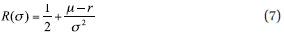Optimal Design Of Risk Control Strategy Indexes

August 17, 2012

Optimal Risk-Control Mechanism
The existing strategies we have discussed so far—leveraged indexes and target volatility indexes—were derived heuristically but are not designed in an optimal way. As we show in this section, both for leveraged indexes and target volatility indexes, there is an optimal way to respond to volatility. Below we discuss the creation of optimal risk-control versions of both methodologies.

Optimally Risk-Controlled Leveraged Indexes
As we have seen in the previous section, leveraged indexes aim to enhance equity returns at the cost of lower Sharpe ratios. The main reason for this is the fact that existing leveraged indexes are not risk controlled.

The model for the expected return of leveraged indexes (5) shows that the rebalancing losses of leveraged indexes increase with equity volatility. Therefore, it makes sense to design leveraged indexes in a risk-controlled way, i.e., they should decrease the level of leverage when volatility goes up and vice versa.

Therefore, in Appendix 4 online, we derive an improved methodology that chooses the optimal way of adjusting the leverage factor (in other words, the size of the equity investment) to changes in volatility. The optimization shows that the optimal way of choosing the leverage factor is given by the following response function, determining the size of the equity investment of the leveraged index:where ?-r denotes the equity risk premium of the underlying equity index. Compared with the target volatility response function (6), the optimally risk-controlled leverage index (7) shows a stronger response to changes in volatility. In particular, it takes more equity risk than the target volatility index in markets of low volatility, as it is a pure return strategy: It does not try to meet a certain volatility level, but aims to maximize returns and takes into account the prevailing level of volatility, in contrast to existing leveraged indexes that are not risk controlled. In Appendix 4 online, we show that using the equity weight (7) creates a leveraged strategy index that has a higher expected return than the underlying equity index, i.e., it is an algorithmic alpha generator, which we will verify by numerical backtests below.

This is a very strong result, as it shows that incorporating a risk-control mechanism into the model framework of leveraged indexes creates strategy indexes that show a much stronger performance than existing strategy indexes in the long run.